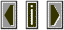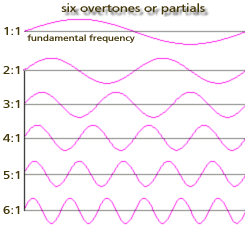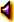PartialThe spectrum of a sound can be reduced to a collection of simple or sinusoidal frequencies.
If these simple frequencies are integer multiples of the fundamental frequency they are said to be in a harmonic relationship and are known as harmonics

All of the signals above the fundamental of the non-sine wave form, includes the harmonics and the inharmonic signals.

Some acousticians reserve the term partial for inharmonic frequency components of the spectrum. These are frequencies which are not integer multiples of the fundamental.

Overtone is another term used to refer to both partials and harmonics.If the simple frequencies pictured in the diagram above are summed together a complex waveform and spectrum will result in the diagram below:first six partialssummed partials